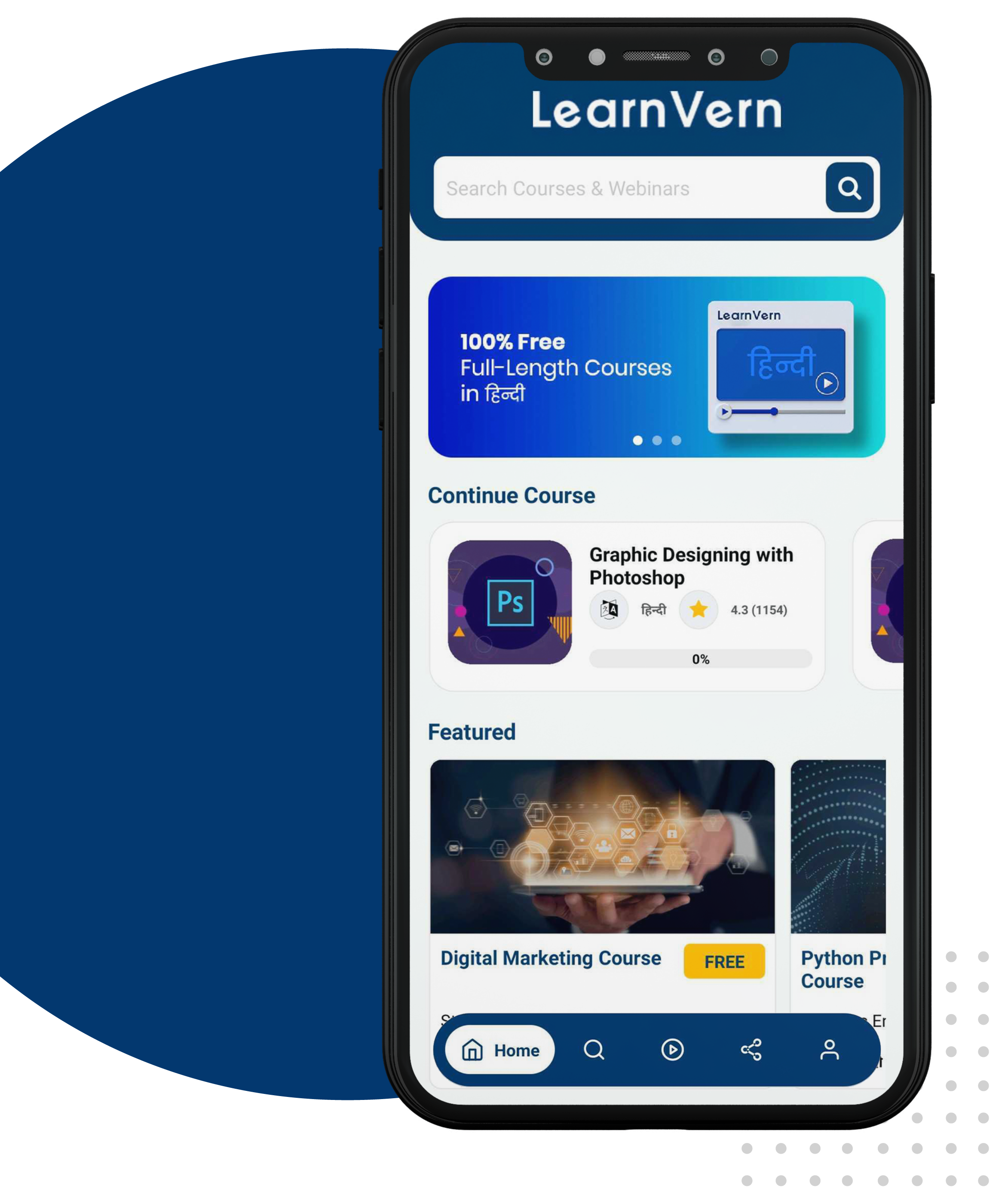If you are facing any issue in playing video please use Firefox browser.

# Description

Python User-defined Functions

In this lecture we will see, How to create and call user defined functions ?

User defined function

User defined function allows you to define functions according to your need. These functions are known as user defined functions. For example, Suppose you need to create a function for finding X power of n.

Writing user defined function in Python -

Step 1 : declare the function with the keyword def followed by the function name.

Step 2 : Write the arguments inside the opening and closing parentheses of the Function, and end the declaration with a colon.

Step 3 : Add the program statements to be executed.

Syntax :

def function_name(argument1, argument2, ...):

statement_1

statement_2

.....

Function with no argument -

def welcome():

print('welcome in python function')

Call a function -

welcome()

Function with one argument -

def sq(x):

print(x**2)

Call a function -

sq(4)

Function with more than one argument -

def power(x,n):

print(x**n)

Call a function -

power(4,3)

Function with return type -

def power(x,n):

return x**n

Call a function -

ans=power(4,3)

print(ans)

Function with multiple return type -

def myfun():

return 1,2,3

Call a function -

a,b,c=myfun()

print('a=',a)

print('b=',b)

print('c=',c)

Example :

def welcome():

print('welcome to my program')

welcome()

O/P -

welcome to my program

Another Example :

def sq(x):

print(x**2)

sq(5)

sq(3)

O/P -

25

9

Another Example :

def power(x,n):

print(x**n)

power(2,5)

O/P -

32

Another Example :

def myfun():

return 1,2,3

a,b,c=myfun()

print('a=',a)

print('b=',b)

print('c=',c)

O/P -

a= 1

b= 2

c= 3

# Share With Friend

Have a friend to whom you would want to share this course?

# Download The Apps###### Code Scan or Download the app###### 297K+ Downloads###### 4.5 Rating###### 10K+ Reviews• Learn anywhere on the go
• Get regular updates about your enrolled or new courses
• Share content with your friends
• Evaluate your progress through practice tests
• No internet connection needed
• Enroll for the webinar and join at the time of the webinar from anywhere